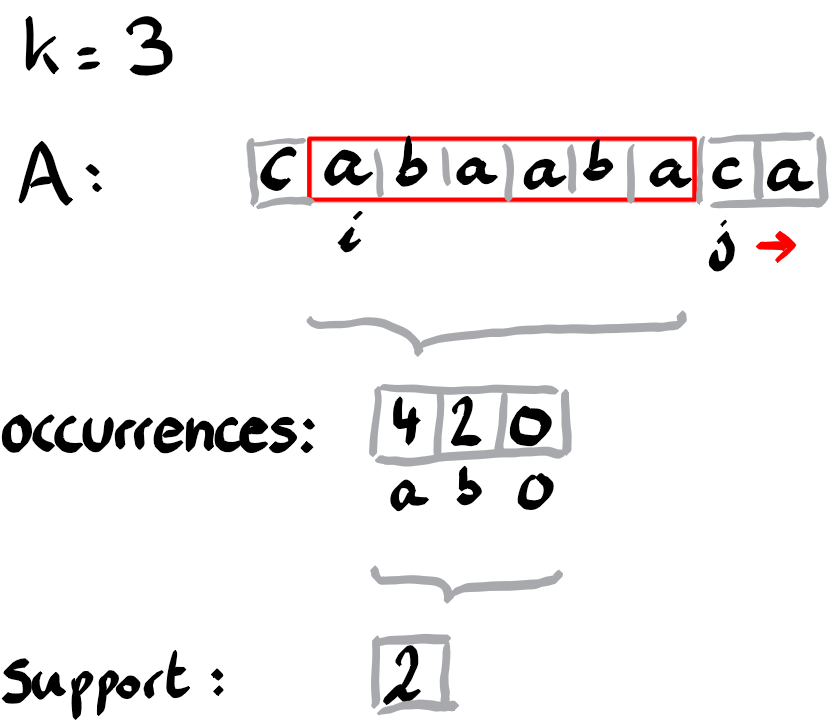# Résolution de problèmes algorithmiques

Given a string A (or array), and an integer k, find for every index i, the smallest index j (if it exists) such that {A[i],..,A[j-1]} consists of exact k distinct values.

# The sliding window technique

The sliding window technique is a simple but versatile technique to solve some problems concerning arrays or strings. By a window we mean an interval on the indexes of the array. Using half open intervals of the form [i,j)={i,i+1,…,j-1} is convenient for this technique. You can answer in linear time questions like, finding the smallest interval containing all distinct values of the array, or counting the number of intervals containing k distinct values. In this post we describe how to find for every i the smallest j such that A[i:j] contains exactly k values. We store this value in a table entry B[i] and write B[i]=-1, if such a value does not exist.

The idea of the sliding window technique is that you maintain some data about the elements in the window. And according to some condition, you either shrink the window (by incrementing the left border) or you grow the window (by incrementing the right border). Let’s see this technique in detail.

# Linear time algorithm

The idea is to maintain a sliding window in the form of an interval [i,j) along the array A. If the interval contains k distinct elements, then we increment i, and if it is missing some elements, we increment j. This way we compute for every i, the smallest j such that [i,j) contains exactly k elements.

In order to check how many distinct elements there are in the current window, we maintain a counter dictionary occurrences indexed by elements, such that occurrences[z] is the number of elements z among A[i:j]. In addition we maintain a variable support, counting the number of elements z such that occurrences[z] is positive.Bookkeeping is fairly straightforward. Before increasing i, decrease occurrences[A[i]]. If that becomes zero, then decrease support as well. Before increasing j, increase occurrences[A[j]]. If it was zero, then increase support as well. Now keep track of the smallest j for every i such that the support has the required size.

# Complexity

This algorithm is fairly efficient, since i+j increases in each iteration and ranges from 0 to 2n at most, where n is the size of A. So the overall complexity is O(n).

# Technical details

Using the C++ post increment and pre increment syntax, one can produce a rather compact code. I like it, but of course the usage of compact instructions is a matter of taste and habit.